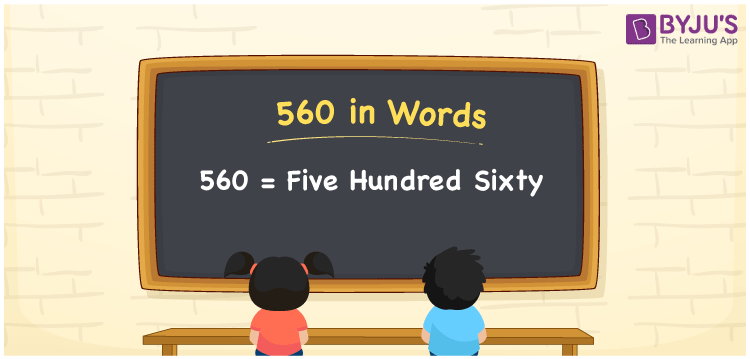# 560 in Words

The number 560 in English is written as Five Hundred Sixty.  560 can be easily converted to word form using a place value chart. Hence, a place value chart is needed to represent the number names. For example, if you bought dry fruits worth Rs. 560, you can say, “I bought dry fruits worth Rupees Five Hundred Sixty”. We know that 560 is a cardinal number as it depicts a specific quantity.

 560 in Words Five Hundred Sixty Five Hundred Sixty in numerical form 560

## 560 in English Words

Generally, we write numbers in words using the English alphabet. It is necessary to learn the English language to grasp and express numbers in a better way. Hence, 560 in words is written as Five Hundred Sixty.## How to Write 560 in Words?

Place value charts help us to write the numbers in words. The below table shows the place value chart for the number 560.

 Hundreds Tens Ones 5 6 0

Therefore,we can write the expanded form as:

5 x Hundred + 6 x Ten + 0 x One

= 5 x 100 + 6 x 10 + 0 x 1

= 500 + 60 + 0

= 500 + 60

= 560

= Five Hundred Sixty

Hence, 560 in words is written as Five Hundred Sixty.

Interesting way of writing 560 in words

5 = Five

56 = Fifty-Six

560 = Five Hundred Sixty

Thus, the word form of the number 560 is Five Hundred Sixty

560 is a natural number that is the successor of 559 and the predecessor of 561

• 560 in words – Five Hundred Sixty
• Is 560 an odd number? – No
• Is 560 an even number? – Yes
• Is 560 a perfect square number? – No
• Is 560 a perfect cube number? – No
• Is 560 a prime number? – No
• Is 560 a composite number? – Yes

## Frequently Asked Questions on 560 in Words

Q1

### How to write 560 in words?

560 in words is written as Five Hundred Sixty.
Q2

### Simplify 400 + 160, and express in words.

Simplifying 400 + 160, we get 560. Thus, 560 in words is written as Five Hundred Sixty.
Q3

### 560 is a prime number. True or False.

False, 560 is not a prime number.